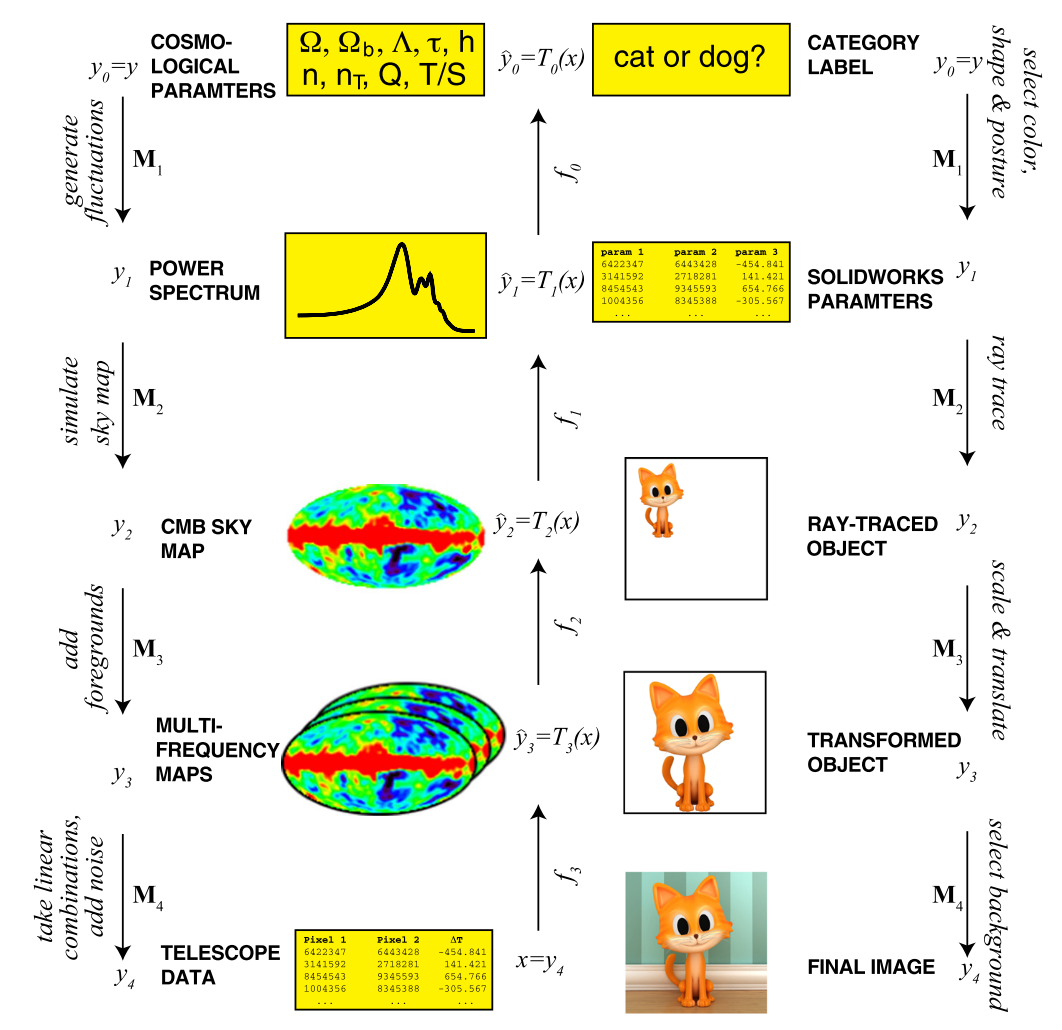# Why does deep and cheap learning work so well?

Lin et al., 2017Source: Lin et al., 2017

## Summary

• How do properties frequently encountered in physics (e.g. symmetry, locality, compositionality) translate into simple neural networks?
• Show that when data is generated from a common hierarchical form, a deep neural network can be more efficient
• Prove various “no-flattening theorems”
• Links: [ website ] [ pdf ]

## Background

• While the universal approximation theorems prove that neural networks can approximate arbitrary functions well, they do little to explain the empirical efficiency of deep networks
• Factors that affect quality of neural networks:
• Expressibility: What class of functions can be expressed?
• Efficiency: How many resources are required to approximate a given function?
• Learnability: How rapidly can the network learn good parameters?
• Focuses on expressibility and efficiency by addressing:

How can neural networks approximate functions well in practice, when the set of possible functions is exponentially larger than the set of practically possible networks?

• The fact that networks of feasible size are useful implies the class of functions we care about is dramatically smaller than all possible functions

## Methods

• Interested in probability distribution $p(\textbf{x}|y)$, where $\textbf{x}$ might be the pixels in an image and $y$ the object label (e.g. cat)
• Probabilities and Hamiltonians
• Hamiltonian: $H_y(\textbf{x}) \equiv -\ln p(\textbf{x}|y)$
• After some algebra (see paper), $p(\textbf{x}) = \frac{1}{N(\textbf{x})}e^{-\left[\mathbf{H(x)+\mu}\right]}$
• Investigate how well $p(\mathbf{x})$ can be approximated by a neural network
• $p(\mathbf{x})$ can be obtained by applying softmax layer to $\mathbf{H(x)}$, therefore question becomes how to approximate $\mathbf{H(x)}$ well

## Results

• Nature favors Hamiltonians that are low-order, sparse, symmetric polynomials – which require few continuous parameters to represent
• Polynomials can be built using multiplications and additions, multiplication can be achieved with four neurons
• Low polynomial order: typically degree ranging from 2 to 4
• Locality: things are only affected by what’s in their immediate vicinity, results in sparsity
• Symmetry: invariance under some transformation (e.g. tranlation or rotation), results in fewer independent parameters
• Why deep?
• No-flattening Theorems: Inability of neural networks representing the data from generative processes with hierarchical structure to be efficiently flattened
• E.g. how matrix multiplication can be optimized by factoring
• Result of the generative process contains much more information than the actual process
• E.g. “generate a random string of $10^9$ bits”

## Conclusion

• Success of reasonably sized neural networks hinges on symmetry, locality, and polynomial log-probability in data from the natural world